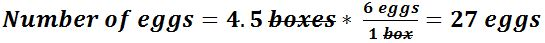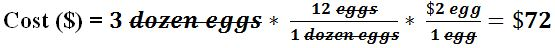# Unit conversions in chemistry

### Unit conversions in chemistry

#### Lessons

In this lesson, we will learn:
• The units of measurements commonly used in chemistry.
• How to use the unit conversion method and the reason it is valuable.
• Practical examples of using the unit conversion method to do calculations in chemistry.

Notes:
• In any problem where information you have has different units to the information you're being asked for, you'll need to do a unit conversion.
• Chemistry calculations involve units like number of moles (units: mol), the mass of a substance (units: g), the volume of a gas, liquid or solution (units: L) and others.
• Calculations in chemistry can be solved by breaking down questions into segments:
• An unknown quantity to be found - the answer to the question.
• An initial quantity to be converted into the units of the unknown quantity.
• A conversion factor(s) linking the unknown quantity and the initial quantity.
• A conversion factor is an expression as a fraction that equates one unit to another. For example: $\frac{1\;min}{60\;s}$ and $\frac{60\;s}{1\;min}$
• Because the value of both terms in the unit conversion are equal (60 seconds is equal to 1 minute), when multiplying by a unit conversion the value of the expression doesn't change.
• This also means you can arrange either term (seconds or mins) on the top or the bottom; arrange it so that your original units cancel and you convert to the new units. This is why it is known as a conversion factor.
• CONVERSION FACTORS WILL CHANGE THE UNITS WITHOUT CHANGING THE VALUE!
• To solve calculations using the unit conversion method, the following steps should be done in order:
• Identify the unknown quantity to be found – this should be written with units and put one side of an equation.
• Identify the initial quantity the question has given you – this starts, with units, on the other side of the equation.
• Apply the unit conversion(s) by multiplying it with the initial quantity you were given.
• This works even if multiple unit conversions are necessary – this method also encourages you to display your working clearly so any mistakes are usually easy to spot!
• For example: If there are 6 eggs in a box, how many eggs would be in 4.5 boxes?
•• For example (part 2): If an egg costs $2 each, how much does 3 dozen eggs cost? •• This method can be used beyond chemistry to solve any problem involving a known quantity that can be converted into another unknown quantity. • Introduction Introduction to unit conversions a) Units and calculations in chemistry. b) What is a unit conversion? c) Unit conversion method: Walkthrough • 1. Apply the conversion factor method to simple calculations. Use the unit conversion method to answer the following problems. a) If a car can travel 75 kilometres in 1 hour, how far can it travel in 4.5 hours? b) An electronics store has an offer that sells two TVs for$430. How much would 8 TVs cost using this deal?

c)
At a market, a man traded 8 apples for 14 oranges. How many oranges can he get if he traded 22 apples at this rate?

• 2.
Apply the conversion factor method to chemistry-related calculations.
Use the unit conversion method to answer the following problems.
a)
If a container holds 3.5 dozen oxygen atoms, how many oxygen atoms in total will 3 full containers have in them?

b)
If one mole of oxygen gas has a mass of 32 g, what is the mass of 3.5 moles of oxygen gas?

c)
If one molecule of white phosphorus has 4 atoms of phosphorus in it, how many molecules of white phosphorus would be needed to have 72 atoms of phosphorus?

d)
If one mole of hydrogen gas fills up 22.4 L in a gas canister, how many moles of hydrogen gas would fill up a gas canister 180 L in size?

• 3.
Apply the conversion factor method to chemistry-related calculations with SI units.
Use the unit conversion method to answer the following problems.
a)
4.7 moles of carbon dioxide gas has a mass of 206.8 g. What is the mass of 1 mole of carbon dioxide gas?

b)
Gold has a density of 19.3 grams per millilitre (g/mL), what would the volume be of 62 grams of gold?

c)
If an acid has a concentration of 3 moles per litre, how many litres of acid would I need to have 1.8 moles of acid?